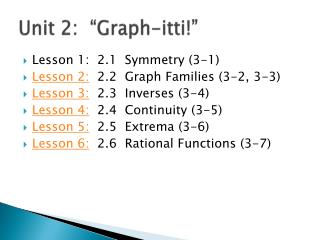# Unit 2: “Graph- itti !” - PowerPoint PPT PresentationDownload PresentationUnit 2: “Graph- itti !”

Unit 2: “Graph- itti !”Download Presentation## Unit 2: “Graph- itti !”

- - - - - - - - - - - - - - - - - - - - - - - - - - - E N D - - - - - - - - - - - - - - - - - - - - - - - - - - -
##### Presentation Transcript

1. Unit 2: “Graph-itti!” • Lesson 1: 2.1 Symmetry (3-1) • Lesson 2: 2.2 Graph Families (3-2, 3-3) • Lesson 3: 2.3 Inverses (3-4) • Lesson 4: 2.4 Continuity (3-5) • Lesson 5: 2.5 Extrema (3-6) • Lesson 6: 2.6 Rational Functions (3-7)

2. Warm-up:

3. Unit Two: “Graph-itti” In this unit we will learn… • STANDARD 2.1: use algebraic tests to determine symmetry in graphs, including even-odd tests (3-1) • STANDARD 2.2: graph parent functions and perform transformations to them (3-2, 3-3) • STANDARD 2.3: determine and graph inverses of functions (3-4) • STANDARD 2.4: determine the continuity and end behavior of functions (3-5) • STANDARD 2.5: use appropriate mathematical terminology to describe the behavior of graphs (3-6) • STANDARD 2.6: graph rational functions (3-7)

4. STANDARD 2.1: use algebraic tests to determine symmetry in graphs, including even-odd tests (3-1) • In this lesson we will… • Discuss what symmetry is and the different types that exist. • Learn to determine symmetry in graphs. • Classify functions as even or odd.

5. What is Symmetry? • Point Symmetry: Symmetry about one point • Figure will spin about the point and land on itself in less than 360º.

6. Formal Definition: P’ M P

7. Symmetry to Origin: • This is the main point we look at for symmetry. • Let’s build some symmetry!

8. Determining Symmetry with Respect to the Origin

9. Let’s do a couple…

10. Line Symmetry

11. Lines We Are Interested In… • x-axis • y-axis • y = x • y = -x

12. x-axis

13. y-axis

14. y = x

15. y = -x

16. Checking Mathematically:

17. Homework: • HW 2.1: P 134 #15 – 35 odd

18. Warm-up: • Get a piece of graph paper and a calculator. • Graph the following on separate axii:

19. Homework:

20. STANDARD 2.2: graph parent functions and perform transformations to them (3-2) In this section we will… • Identify the graphs of some simple functions. • Recognize and perform transformations of simple graphs. • Sketch graphs of related functions.

21. Families of Graphs: • Any function based on a simple function will have the basic “look” of that family. • Multiplying, dividing, adding or subtracting from the function may move it, shrink it or stretch it but won’t change its basic shape.

22. Example:

23. Reflections

24. Vertical Translations

25. Horizontal Translations

26. Vertical Dilations

27. Horizontal Dilations

28. Let’s do some… Send One person from your group to get a white board with a graph on it, a pen and an eraser.

29. STANDARD 2.2: graph parent functions and perform transformations to them (3-3) In this section we will… • Use function families to graph inequalities.

30. So… how do I graph this?

31. Homework: • HW1 2.2: P 143 #13-29 odd, 33 • HW2 2.2: P 150 #21-31 odd

32. Warm-up:

33. Homework:

34. STANDARD 2.3: determine and graph inverses of functions (3-4) In this section we will… • Determine inverses of relations and functions. • Graph functions and their inverses.

35. Inverse Relations: • An inverse of function will take the answers (range) from the function and give back the original domain.

36. Finding the inverse of a relation: • Easy!!! Just switch the domain and range! • Are they both functions?

37. Property of Inverse Functions: • If f(x) and f –1(x) are inverse functions, then • In other words… • Two relations are inverse relations iff one relation contains the element (b,a) whenever the other relation contains (a,b). • Does this remind you of something?

38. Let’s look at them on a graph:

39. A function and its inverse… • Are reflections of each other over the line y = x.

40. Is the inverse a function?

41. Quick and dirty test: • If the original function passes the HORIZONTAL line test then the inverse will be a function. • Let’s check our parent graphs.

42. Is the inverse a function?

43. Is this a function?

44. Proving Inverses: • If two functions are actually inverses then both the composites of the functions will equal x. • You must prove BOTH true.

45. Check to see if the following are inverse functions:

46. The Handy, Dandy Build Your Own Inverse Kit: • Replace f(x) with y (it is just easier to look at this way). • Switch the x and y in the equation. • Resolve the equation for y. • The result is the inverse. • Now check!

47. Try this…

48. Word Problem: • The fixed costs for manufacturing a particular stereo system are \$96,000, and the variable costs are \$80 per unit. • A. Write an equation that expresses the total cost C(x) as a function of x given that x units are manufactured.